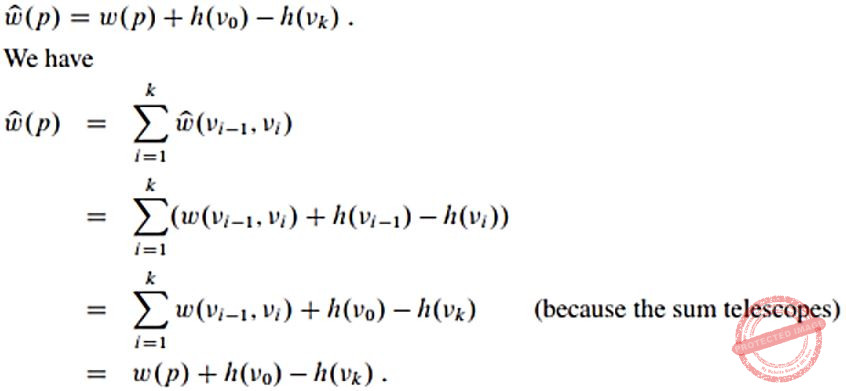October 10, 2023
October 10, 2023
October 10, 2023
###### Software-Engineering
October 10, 2023
 Question 7

Let G = (V,E) be a directed, weighted graph with weight function w:E → R. For some function f:V → R, for each edge (u,v) ∈ E, define w'(u,v) as w(u,v) + f(u) – f(v).
Which one of the options completes the following sentence so that it is TRUE?

“The shortest paths in G under w are shortest paths under w’ too, _______”.

 A if and only if f(u) is the distance from s to u in the graph obtained by adding a new vertex s to G and edges of zero weight from s to every vertex of G B if and only if ∀u ∈ V, f(u) is positive C if and only if ∀u ∈ V, f(u) is negative D for every f: V→R
Question 7 Explanation:Question 7 Explanation: